# Douglas Rachford Proximal Splitting¶

$\newcommand{\dotp}{\langle #1, #2 \rangle}$ $\newcommand{\enscond}{\lbrace #1, #2 \rbrace}$ $\newcommand{\pd}{ \frac{ \partial #1}{\partial #2} }$ $\newcommand{\umin}{\underset{#1}{\min}\;}$ $\newcommand{\umax}{\underset{#1}{\max}\;}$ $\newcommand{\umin}{\underset{#1}{\min}\;}$ $\newcommand{\uargmin}{\underset{#1}{argmin}\;}$ $\newcommand{\norm}{\|#1\|}$ $\newcommand{\abs}{\left|#1\right|}$ $\newcommand{\choice}{ \left\{ \begin{array}{l} #1 \end{array} \right. }$ $\newcommand{\pa}{\left(#1\right)}$ $\newcommand{\diag}{{diag}\left( #1 \right)}$ $\newcommand{\qandq}{\quad\text{and}\quad}$ $\newcommand{\qwhereq}{\quad\text{where}\quad}$ $\newcommand{\qifq}{ \quad \text{if} \quad }$ $\newcommand{\qarrq}{ \quad \Longrightarrow \quad }$ $\newcommand{\ZZ}{\mathbb{Z}}$ $\newcommand{\CC}{\mathbb{C}}$ $\newcommand{\RR}{\mathbb{R}}$ $\newcommand{\EE}{\mathbb{E}}$ $\newcommand{\Zz}{\mathcal{Z}}$ $\newcommand{\Ww}{\mathcal{W}}$ $\newcommand{\Vv}{\mathcal{V}}$ $\newcommand{\Nn}{\mathcal{N}}$ $\newcommand{\NN}{\mathcal{N}}$ $\newcommand{\Hh}{\mathcal{H}}$ $\newcommand{\Bb}{\mathcal{B}}$ $\newcommand{\Ee}{\mathcal{E}}$ $\newcommand{\Cc}{\mathcal{C}}$ $\newcommand{\Gg}{\mathcal{G}}$ $\newcommand{\Ss}{\mathcal{S}}$ $\newcommand{\Pp}{\mathcal{P}}$ $\newcommand{\Ff}{\mathcal{F}}$ $\newcommand{\Xx}{\mathcal{X}}$ $\newcommand{\Mm}{\mathcal{M}}$ $\newcommand{\Ii}{\mathcal{I}}$ $\newcommand{\Dd}{\mathcal{D}}$ $\newcommand{\Ll}{\mathcal{L}}$ $\newcommand{\Tt}{\mathcal{T}}$ $\newcommand{\si}{\sigma}$ $\newcommand{\al}{\alpha}$ $\newcommand{\la}{\lambda}$ $\newcommand{\ga}{\gamma}$ $\newcommand{\Ga}{\Gamma}$ $\newcommand{\La}{\Lambda}$ $\newcommand{\si}{\sigma}$ $\newcommand{\Si}{\Sigma}$ $\newcommand{\be}{\beta}$ $\newcommand{\de}{\delta}$ $\newcommand{\De}{\Delta}$ $\newcommand{\phi}{\varphi}$ $\newcommand{\th}{\theta}$ $\newcommand{\om}{\omega}$ $\newcommand{\Om}{\Omega}$

This numerical tour presents the Douglas-Rachford (DR) algorithm to minimize the sum of two simple functions. It shows an application to reconstruction of exactly sparse signal from noiseless measurement using $\ell^1$ minimization.

In :
from __future__ import division
%pylab inline

Populating the interactive namespace from numpy and matplotlib


## Douglas-Rachford Algorithm¶

The Douglas-Rachford (DR) algorithm is an iterative scheme to minimize functionals of the form $$\umin{x} f(x) + g(x)$$ where $f$ and $g$ are convex functions, of which one is able to compute the proximity operators.

This algorithm was introduced in

P. L. Lions and B. Mercier "Splitting Algorithms for the Sum of Two Nonlinear Operators," SIAM Journal on Numerical Analysis vol. 16, no. 6, 1979,

as a generalization of an algorithm introduced by Douglas and Rachford in the case of quadratic minimization (which corresponds to solving a positive definite linear system).

Patrick L. Combettes and Jean-Christophe Pesquet, "Proximal Splitting Methods in Signal Processing," in: Fixed-Point Algorithms for Inverse Problems in Science and Engineering, New York: Springer-Verlag, 2010.

The Douglas-Rachford algorithm takes an arbitrary element $s^{(0)}$, a parameter $\ga>0$, a relaxation parameter $0<\rho<2$ and iterates, for $k=1,2,\ldots$ $$\left|\begin{array}{l} x^{(k)} = \mathrm{prox}_{\gamma f} (s^{(k-1)} )\\ s^{(k)} = s^{(k-1)}+\rho\big(\text{prox}_{\ga g}( 2x^{(k)}-s^{(k-1)})-x^{(k)}\big). \end{array}\right.$$

It is of course possible to inter-change the roles of $f$ and $g$, which defines a different algorithm.

The iterates $x^{(k)}$ converge to a solution $x^\star$ of the problem, i.e. a minimizer of $f+g$.

## Compressed Sensing Acquisition¶

Compressed sensing acquisition corresponds to a random projection $y=Ax^\sharp$ of a signal $x^\sharp$ on a few linear vectors (the rows of the matrix $A$). For the recovery of $x^\sharp$ to be possible, this vector is supposed to be sparse in some basis. Here, we suppose $x^\sharp$ itself is sparse.

We initialize the random number generator for reproducibility.

In :
random.seed(0)


Dimension of the problem.

In :
N = 400


Number of measurements.

In :
P = round(N/4)


We create a random Gaussian measurement matrix $A$.

In :
A = randn(P,N) / sqrt(P)


Sparsity of the signal.

In :
S = 17


We begin by generating a $S$-sparse signal $x^\sharp$ with $S$ randomized values. Since the measurement matrix is random, one does not care about the sign of the nonzero elements, so we set values equal to one.

In :
sel = random.permutation(N)
sel = sel[0:S]   # indices of the nonzero elements of xsharp
xsharp = zeros(N)
xsharp[sel] = 1


We perform random measurements $y=Ax^\sharp$ without noise.

In :
y = A.dot(xsharp) # matrix-vector multiplication in Python, more precisely dot product of a 2-D array with a 1-D array.


## Compressed Sensing Recovery with the Douglas-Rachford algorithm¶

Compressed sensing recovery corresponds to solving the inverse problem $y=A x^\sharp$, which is ill posed because $x^\sharp$ is higher dimensional than $y$.

The reconstruction can be performed by $\ell^1$ minimization, which regularizes the problem by exploiting the prior knowledge that the solution is sparse. $$x^\star \in \arg\min_x \norm{x}_1 \quad\mbox{s.t.}\quad Ax=y$$ where the $\ell^1$ norm is defined as $$\norm{x}_1 = \sum_{n=1}^N \abs{x_n}.$$

This is the minimization of a non-smooth function under affine constraints. This can be shown to be equivalent to a linear programming problem, for wich various algorithms can be used (simplex, interior points). We propose here to use the Douglas-Rachford algorithm.

It is possible to recast this problem as the minimization of $f+g$ where $g(x) = \norm{x}_1$ and $f(x)=\iota_{\Omega}$ where $\Omega = \enscond{x}{Ax=y}$ is an affine space, and $\iota_\Omega$ is the indicator function $$\iota_\Omega(x) = \choice{ 0 \qifq x \in \Omega, \\ +\infty \qifq x \notin \Omega. }$$

The proximal operator of the $\ell^1$ norm is soft thresholding: $$\text{prox}_{\gamma \norm{\cdot}_1}(x)_n = \max\pa{ 0, 1-\frac{\ga}{\abs{x_n}} } x_n.$$

In :
def prox_gamma_g (x, gamma) :
return x - x/maximum(abs(x)/gamma,1) # soft-thresholding


Display the 1-D curve of the thresholding.

In :
figsize(9,6)
t = arange(-1,1,0.001)
plot(t, prox_gamma_g(t,0.3))
axis('equal')

Out:
(-1.0, 1.0, -0.80000000000000004, 0.80000000000000004)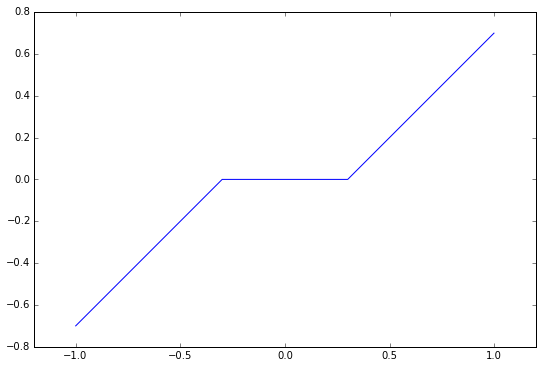The proximity operator of $\gamma$ times the indicator function of $\Omega$ is projection onto $\Omega$ and does not depends on $\gamma$. $$\mathrm{prox}_{\gamma f}(x)=\mathrm{prox}_{\iota_\Omega}(x)=P_\Omega(x) = x + A^* (A A^*)^{-1} (y-Ax).$$

In :
pA = pinv(A) # pseudo-inverse. Equivalent to pA = A.T.dot(inv(A.dot(A.T)))
def prox_f (x, y) :
return x + pA.dot(y-A.dot(x))


We set the values of $\gamma$ and $\rho$. Try different values to speed up the convergence.

In :
gamma = 0.1 # try 1, 10, 0.1
rho = 1     # try 1, 1.5, 1.9


Number of iterations.

In :
nbiter = 700


Exercise

Implement nbiter iterations of the Douglas-Rachford algorithm. Keep track of the evolution of the $\ell^1$ norm.

In :
s = zeros(N)
En_array = zeros(nbiter)
for iter in range(nbiter):  # iter goes from 0 to nbiter-1
En_array[iter] = norm(x, ord=1)
x_restored = x


We display the original and the recovered signals.

In :
fig, (subfig1,subfig2) = subplots(1,2,figsize=(16,7)) # one figure with two horizontal subfigures
subfig1.stem(xsharp)
subfig1.set_ylim(0,1.1)
subfig2.stem(x_restored)
subfig2.set_ylim(0,1.1)
subfig1.set_title('$x^\sharp$')
subfig2.set_title('$x_\mathrm{restored}$')

Out:
<matplotlib.text.Text at 0x109841710>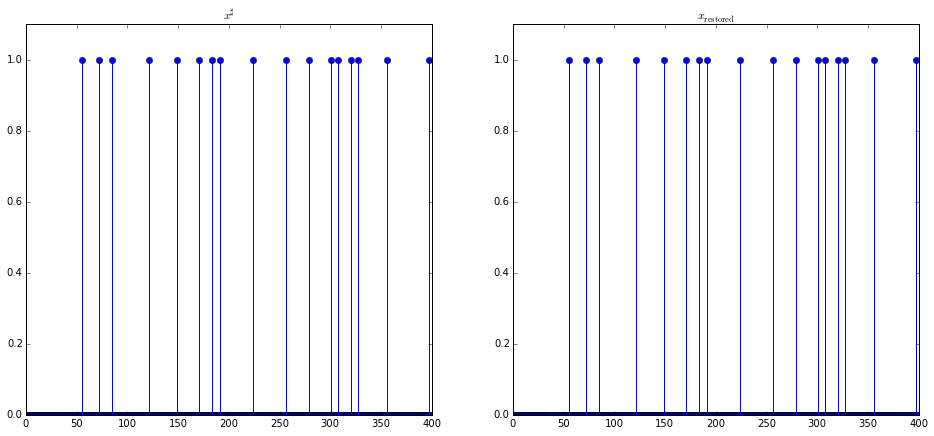Since the original signal is highly sparse, it is perfectly recovered.

We display the convergence speed of the $\ell^1$ norm on the first half iterations, in log scale.

In :
plot(log10(En_array-En_array.min()))

/Users/condatl/anaconda/lib/python2.7/site-packages/IPython/kernel/__main__.py:1: RuntimeWarning: divide by zero encountered in log10
if __name__ == '__main__':

Out:
[<matplotlib.lines.Line2D at 0x10b64a790>]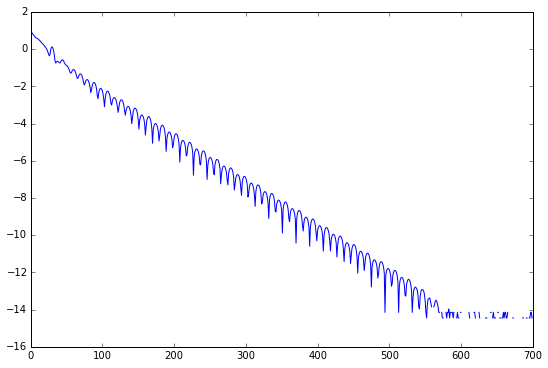The convergence is linear in practice. Convergence up to machine precision (1e-14 here) is achieved after a few hundreds of iterations.

Exercise

Test the recovery of a less sparse signal. What do you observe?

In :
S = 31
random.seed(0)
sel = random.permutation(N)
sel = sel[0:S]   # indices of the nonzero elements of xsharp
xsharp = zeros(N)
xsharp[sel] = 1

y = A.dot(xsharp)


In :
fig, (subfig1,subfig2) = subplots(1,2,figsize=(16,7)) # one figure with two horizontal subfigures
subfig1.stem(xsharp)
subfig1.set_ylim(0,1.1)
subfig2.stem(x_restored)
subfig1.set_title('$x^\sharp$')
subfig2.set_title('$x_\mathrm{restored}$')

Out:
<matplotlib.text.Text at 0x10ba4f050>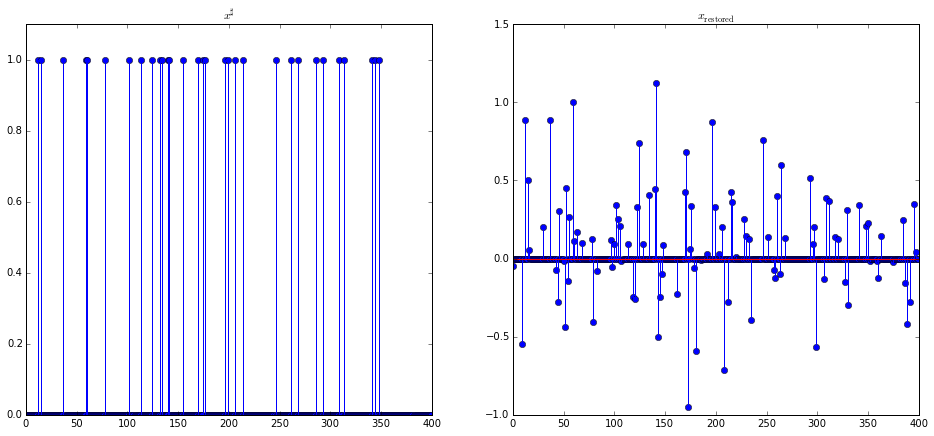In :
plot(log10(En_array-En_array.min()))

/Users/condatl/anaconda/lib/python2.7/site-packages/IPython/kernel/__main__.py:1: RuntimeWarning: divide by zero encountered in log10
if __name__ == '__main__':

Out:
[<matplotlib.lines.Line2D at 0x10cc66950>]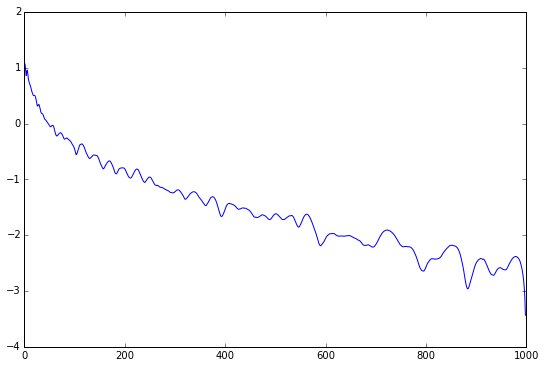Convergence is much slower in this setting.